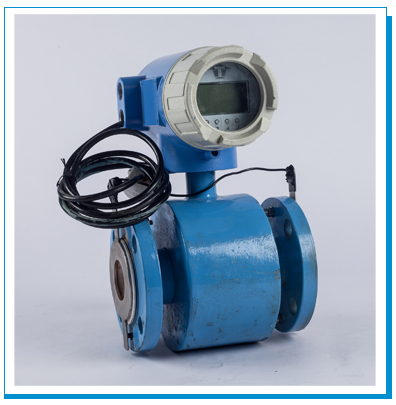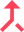Click for Zoom

Electromagnetic flow measurement is based on Faraday's Law of Induction. The law states that voltage is induced across a conductor moving through a magnetic field. The functional principle of electromagnetic measuring devices is also based on this law of nature. An emf is generated across a conductor moving in a magnetic field. This emf is directly proportional to the flux density, velocity of conductor and length of the conductor. This principle is used for flow measurement through electromagnetic flow meter. The flowing liquid itself is a conductor and its average velocity is the velocity of conductor.

E = BYV.D.
Where
E = Induced emf proportional to velocity.
B = Magnetic flux Density
V = Average velocity of the media
D = Distance between two electrodes or Practically the diameter of the flow sensor

Since the Flux Density and diameter of the flow sensor are fixed for given combination Theemf becomes proportional to average velocity only and in turn the volumetric flow rate.

Electromagnetic flow meters are based on the laws of electromagnetic induction, conductive liquids are the only liquids for which flow can be detected. Whether it is a conductive liquid or not is determined by the presence of electrical conductivity. Minimum conductivity of fluid should be 0.5 us/cm